## Selina Concise Mathematics Class 9 ICSE Solutions Pythagoras Theorem [Proof and Simple Applications with Converse]

APlusTopper.com provides step by step solutions for Selina Concise Mathematics Class 9 ICSE Solutions Chapter 13 Pythagoras Theorem [Proof and Simple Applications with Converse]. You can download the Selina Concise Mathematics ICSE Solutions for Class 9 with Free PDF download option. Selina Publishers Concise Mathematics for Class 9 ICSE Solutions all questions are solved and explained by expert mathematic teachers as per ICSE board guidelines.

Selina ICSE Solutions for Class 9 Maths Chapter 13 Pythagoras Theorem [Proof and Simple Applications with Converse]

Exercise 13(A)

Solution 1:Solution 2: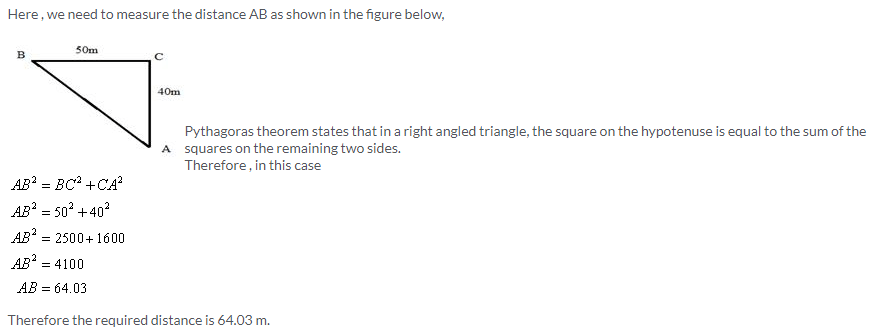Solution 3: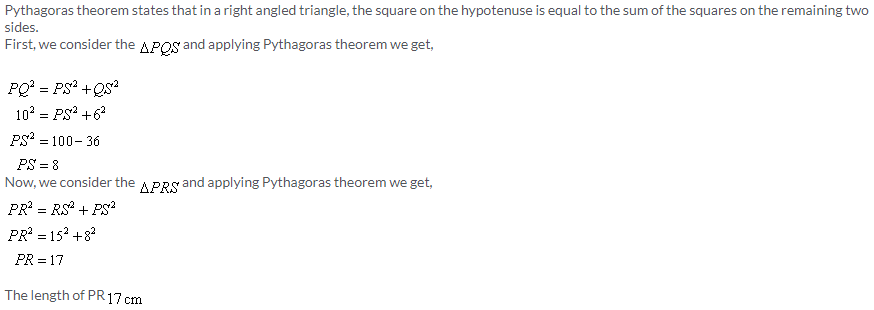Solution 4:Solution 5:Solution 6: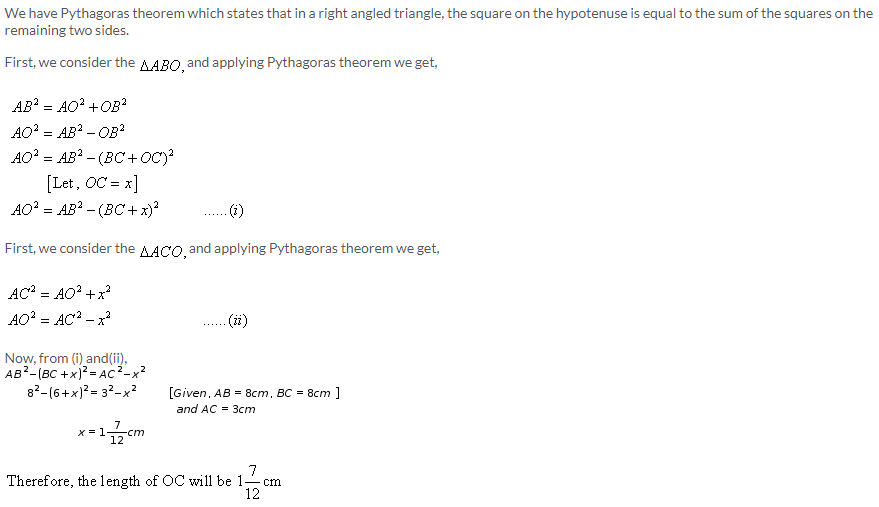Solution 7: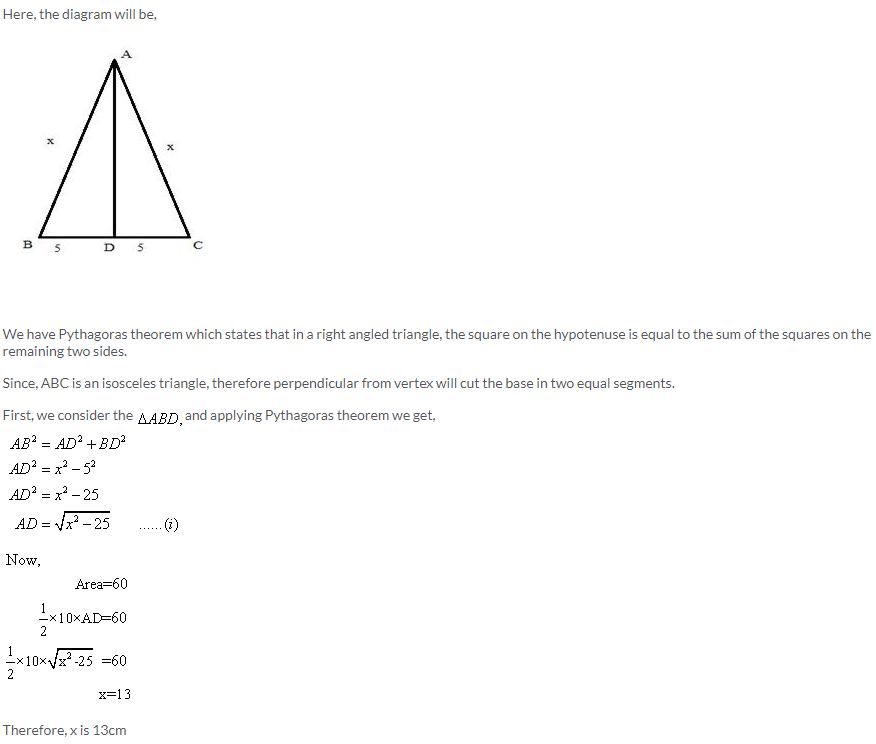Solution 8: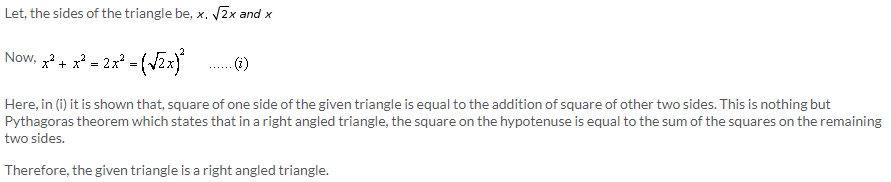Solution 9: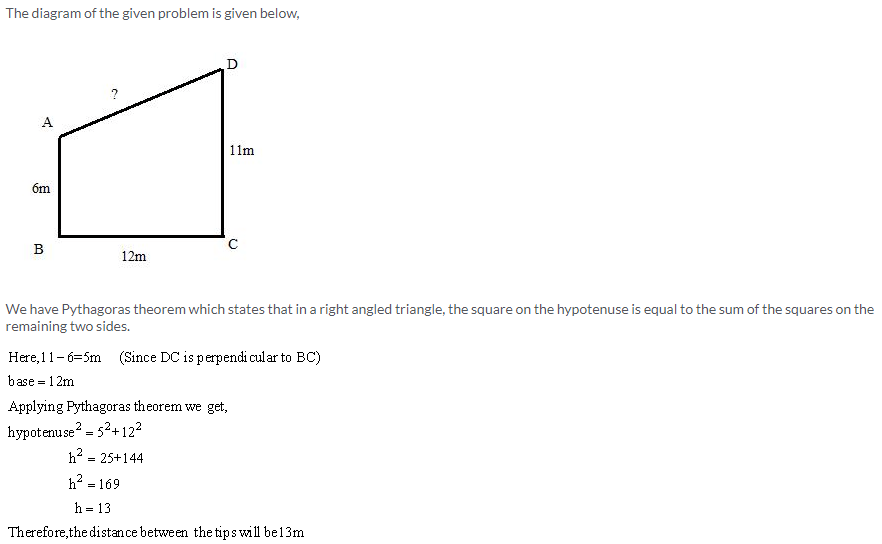Solution 10:
Take M be the point on CD such that AB = DM.
So DM = 7cm and MC = 10 cm
Join points B and M to form the line segment BM.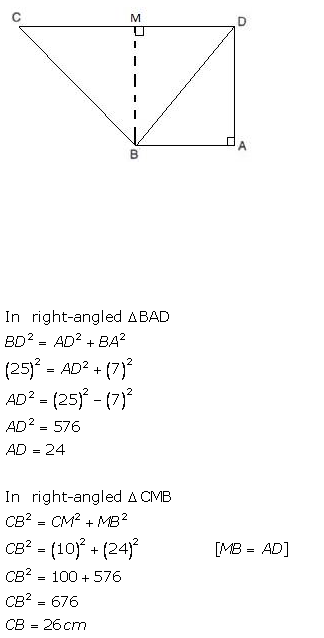Solution 11:
Given that AX:XB = 1:2.
Let n be the common multiple for which this proportion gets satisfied.
So, AX = 1(n) and XB = 2(n)AX = 1(n) = 4 and XB = 2(n) = 8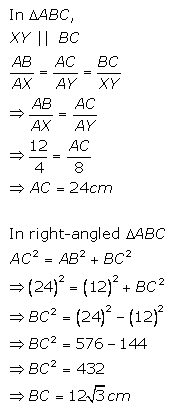Solution 12: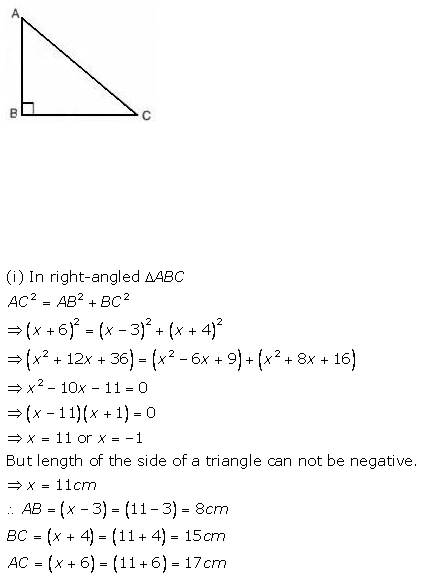Exercise 13(B)

Solution 1: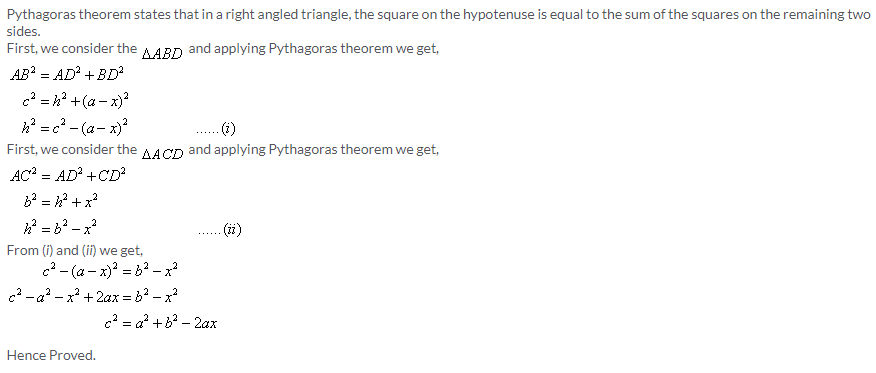Solution 2:Solution 3: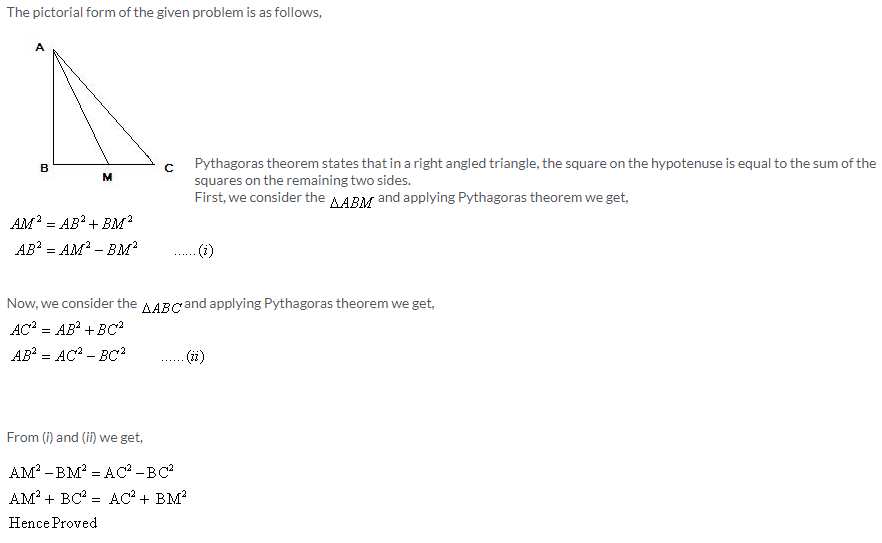Solution 4: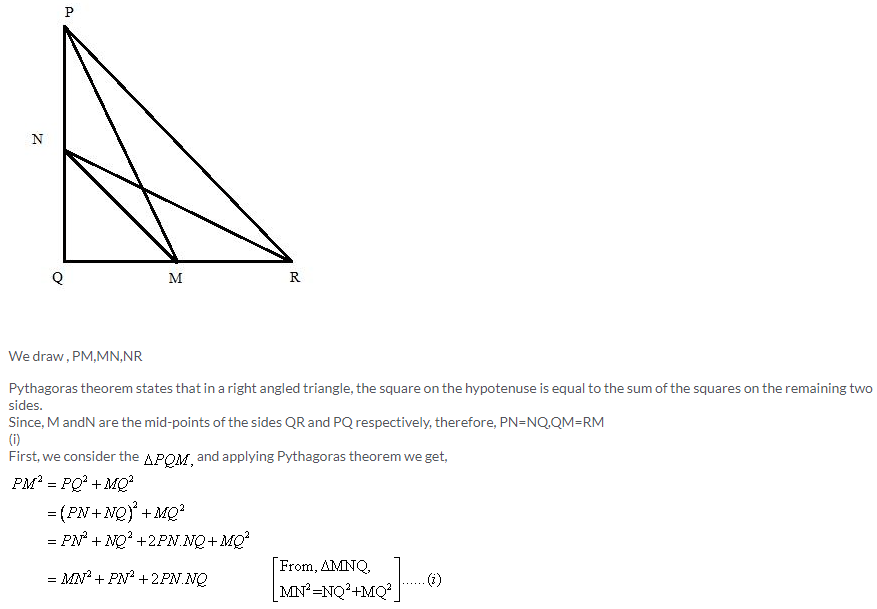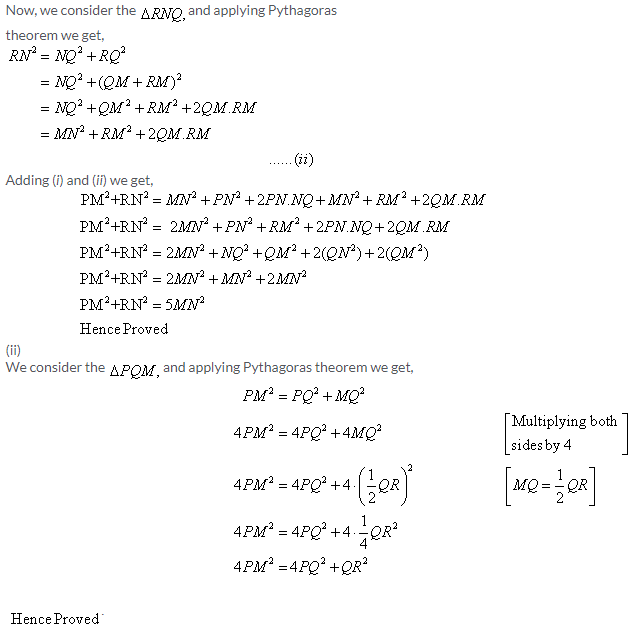Solution 5: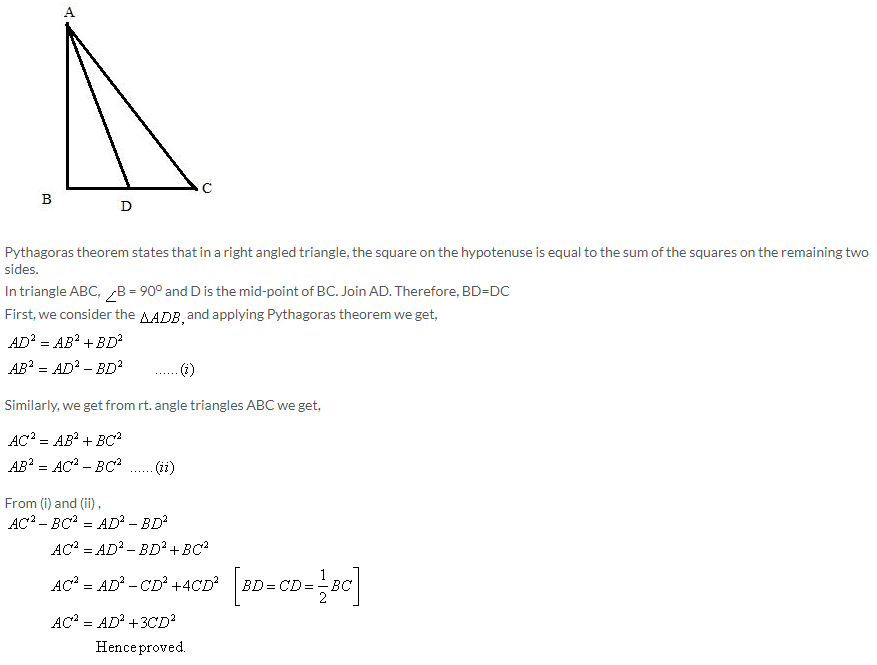Solution 6:Solution 7:Solution 8:Solution 9: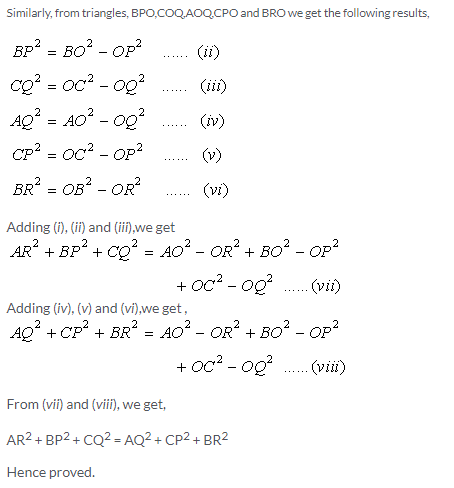Solution 10: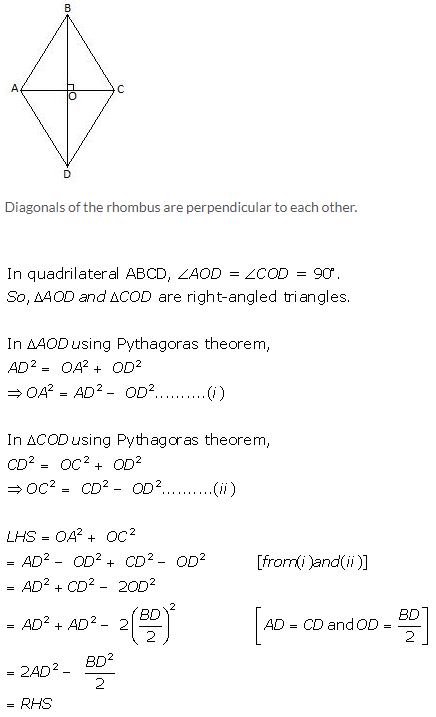Solution 11:Solution 12: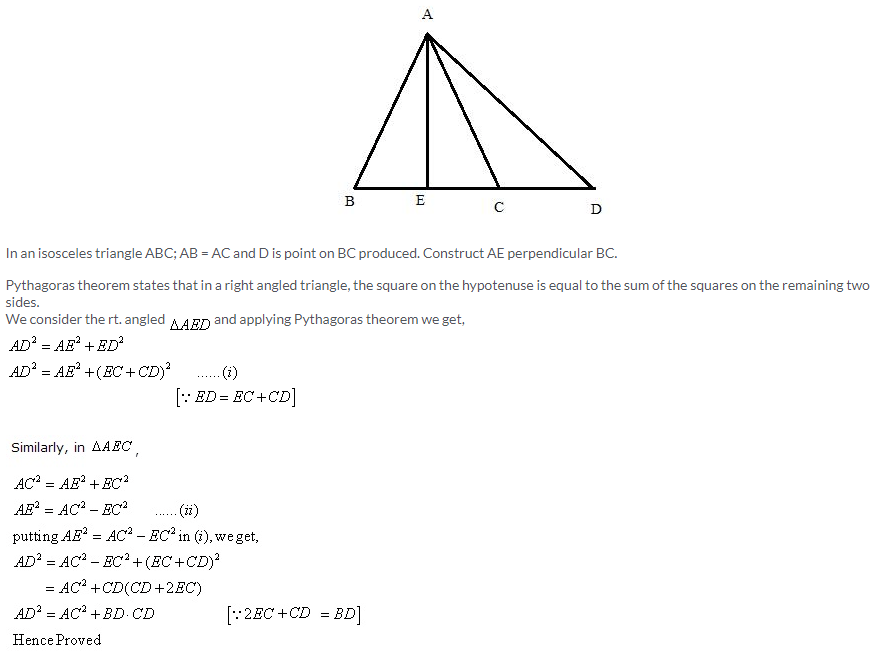Solution 13:Solution 14: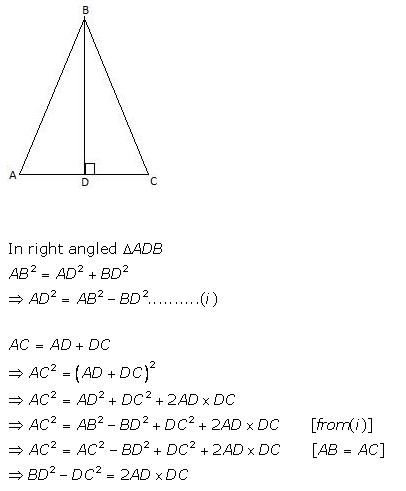Solution 15: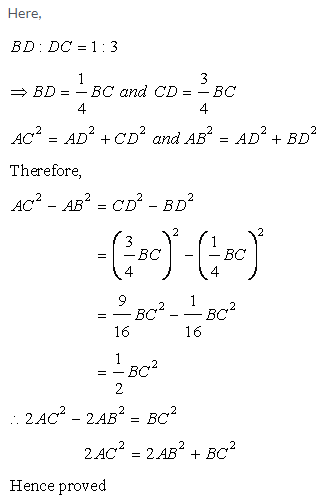More Resources for Selina Concise Class 9 ICSE Solutions